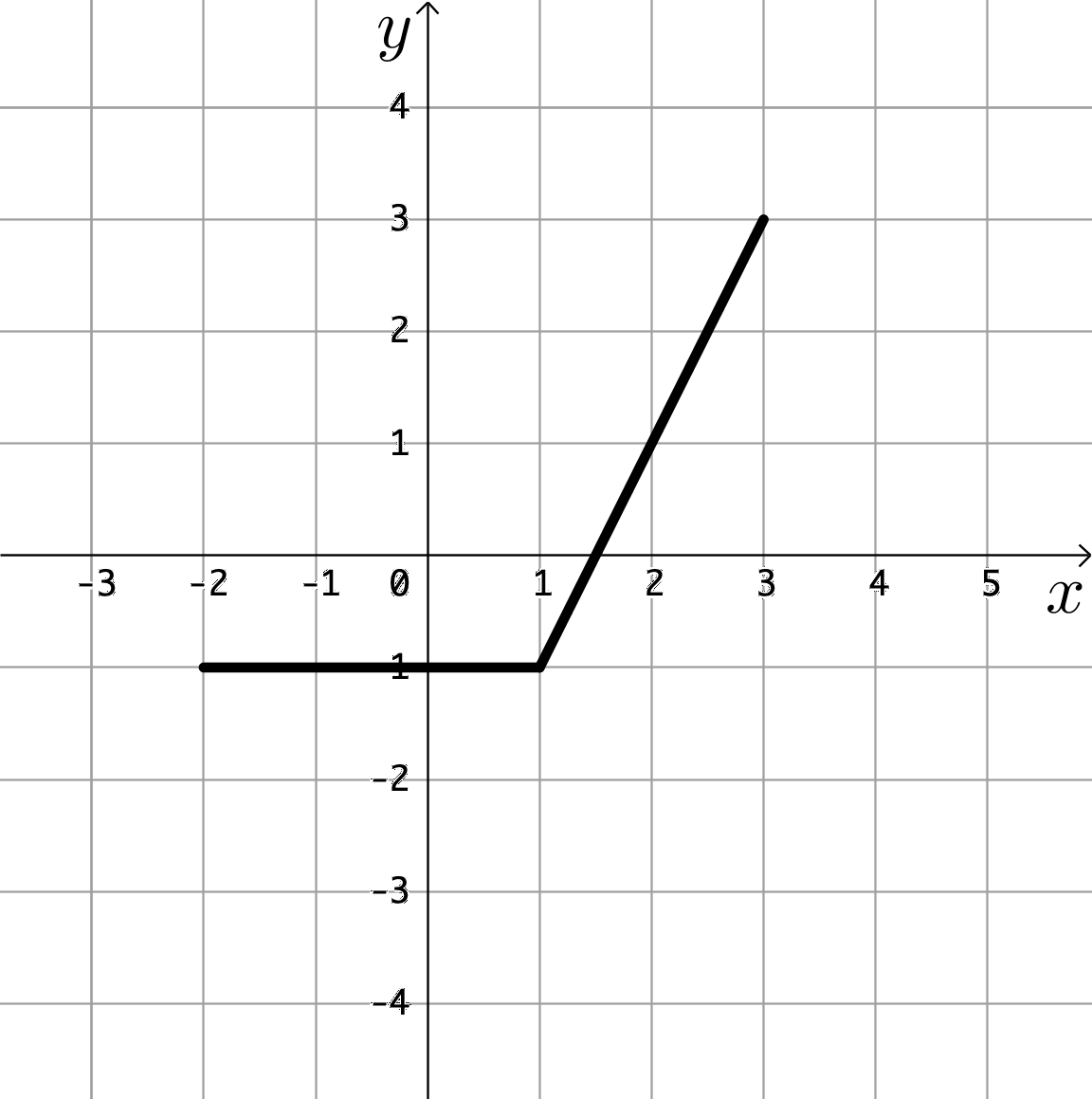$\newenvironment {prompt}{}{} \newcommand {\ungraded }{} \newcommand {\HyperFirstAtBeginDocument }{\AtBeginDocument }$

Determine an antiderivative for $f(x)=\cos (x^2)$
$2x \sin (x^2)$ $-\sin \left ( \frac {x^3}{3} \right )$ $\int _0^x \cos (t^2) dt$ $\int _0^x 2t \sin (t^2) dt$ $\int _0^x -\sin \left ( \frac {t^3}{3} \right ) dt$ $f(x)$ doesn’t have an antiderivative
If $F(x) = \int _0^x \cos (e^t) dt$, what is $F’(x)$? $\answer {\cos (e^x)}$
$F(x) = \int _0^x -4t+12 dt$. On the interval $[0,4]$, $F(x)$ is:
The following graph is of $y = f(t)$ over the interval $[-2,3]$. Which of the following choices could be the corresponding graph of $F(x) = \int _{-2}^x f(t) dt$?
 Graph aGraph bGraph cGraph dGraph eGraph fGraph gGraph h